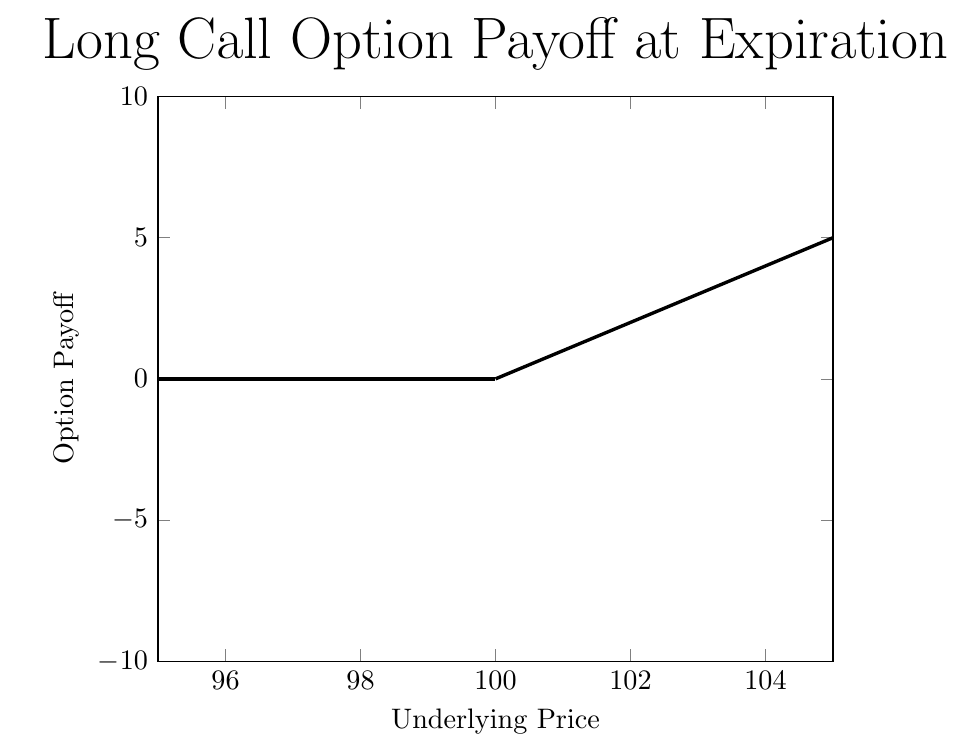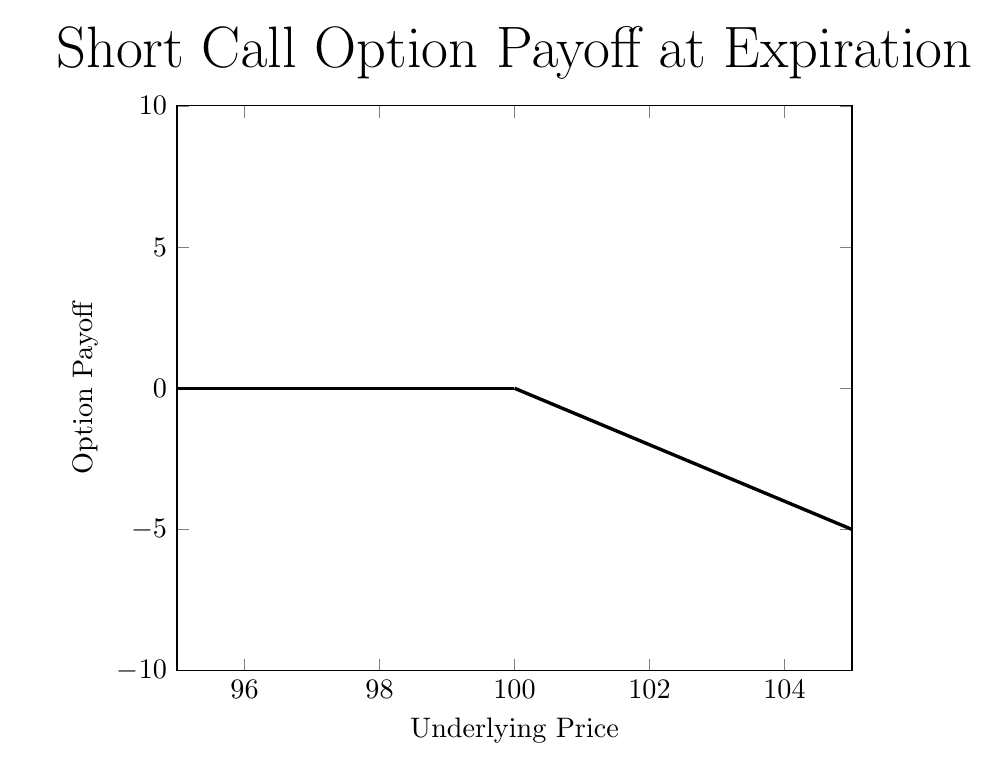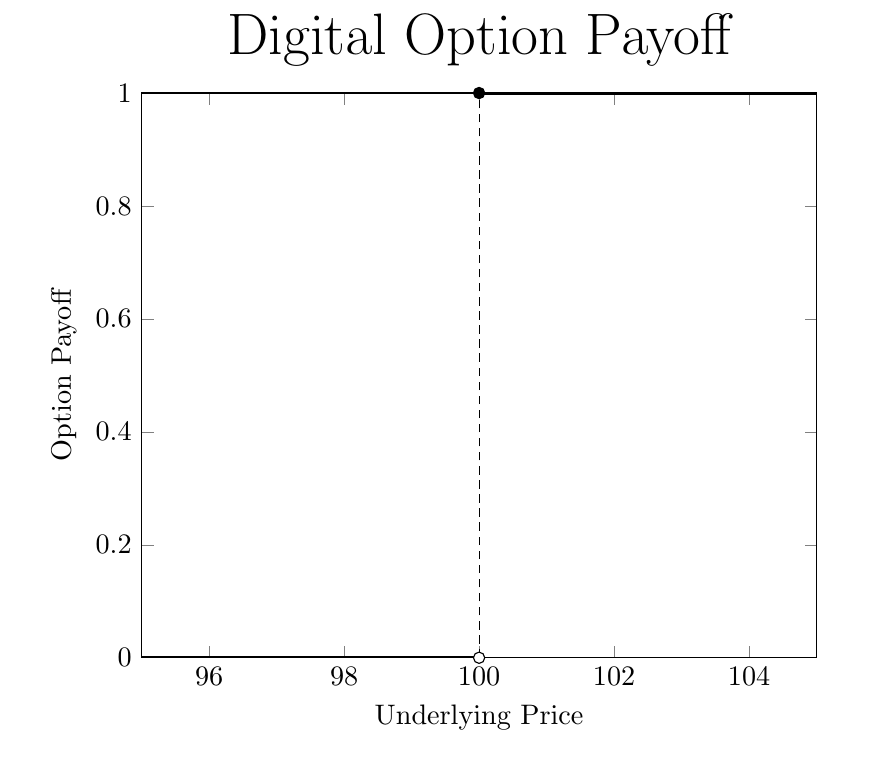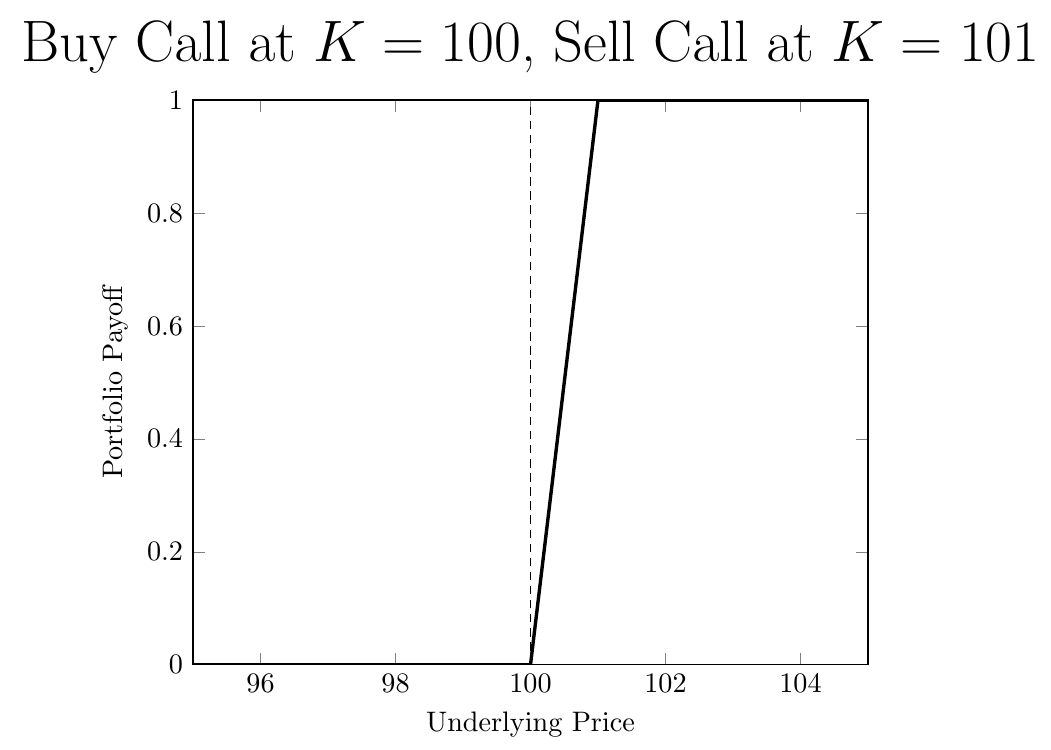This post is based on problems 2.10 and 2.11 in, “Heard on the Street” by Timothy Falcon Crack. I was asked how to price a digital option in a job interview - and had no idea what to do!

# European Call Options

A European call option is the right to buy an asset at the strike price, $$K$$, on the option’s expiration date, $$T$$. A call is only worth exercising (using) if the underlying price, $$S$$, is greater than $$K$$ at $$T$$, as the payoff from exercising is $$S-K$$. The plot below shows the value of a call option, as a function of the underlying asset’s price, with $$K=100$$:Selling a call option with a strike $$K=100$$ earns you the call’s price, $$c$$, today, but your payoff will be decreasing in the underlying price:# Digital Call Options

A digital call option with $$K=100$$ is similar - it pays off one dollar if $$S\geq100$$ at expiration, and pays off zero otherwise:Suppose you have a model for pricing regular call options. If you’re using Black-Scholes the price of the call, $$c$$, is a function of $$K$$, $$S$$, time to expiration $$T-t$$, the volatility of the underlying asset $$\sigma$$, and the risk free rate $$r$$: \begin{equation} c=F(K,S,T-t,\sigma,r) \end{equation} Now - suppose the model is correct. How can you use $$F(K,\cdot)$$ to price the digital option?

# Replicating the Digital Option

The trick is to replicate the digital option’s payoff with regular calls. As a starting point, consider buying a call with $$K=100$$ and selling a call with $$K=101$$:This is close to the digital option, but not exactly right. We want to make the slope at 100 steeper, so we need to buy more options. This is because a call’s payoff increases one-for-one with the underlying once the option is in the money, so with one option you are stuck with a slope of one.
Consider buying two calls with $$K=100$$ and selling two calls at $$K=100.5$$:As opposed to a slope of 1 between 100 and 101, now we have a slope of two between 100 and 100.5.

Generalizing this idea - consider a number $$\epsilon>0$$. To get a slope of $$\frac{1}{\epsilon}$$, you buy $$\frac{1}{\epsilon}$$ calls at $$K=100$$ and you sell $$\frac{1}{\epsilon}$$ calls at $$K=100+\epsilon$$. Here’s what it looks like for $$\epsilon=\frac{1}{10}$$:Given that the slope is $$\frac{1}{\epsilon}$$, to get an infinite slope, we take the limit as $$\epsilon$$ goes to zero.

How much will the above portfolio cost? You earn $$\frac{1}{\epsilon}F(100+\epsilon, \cdot)$$ from selling the $$K=100+\epsilon$$ calls, and pay $$\frac{1}{\epsilon}F(100, \cdot)$$ for the $$K=100$$ calls. The net cost is: \begin{equation} lim_{\epsilon \rightarrow 0} \frac{F(100+\epsilon,\cdot)-F(100,\cdot)}{\epsilon} \end{equation}

What does this look like? A derivative! It might look more familiar if I re-wrote it as:

\begin{equation} lim_{\epsilon \rightarrow 0} \frac{F(K+\epsilon)-F(K)}{\epsilon} \end{equation}

The price of the digital option is the derivative of $$F$$ with respect to the strike price $$K$$.

# Conclusion

Many complicated payoffs can be re-created as combinations of vanilla puts and calls. For an overview, see the first few chapters of Sheldon Natenberg’s, “Option Volatility & Pricing”.